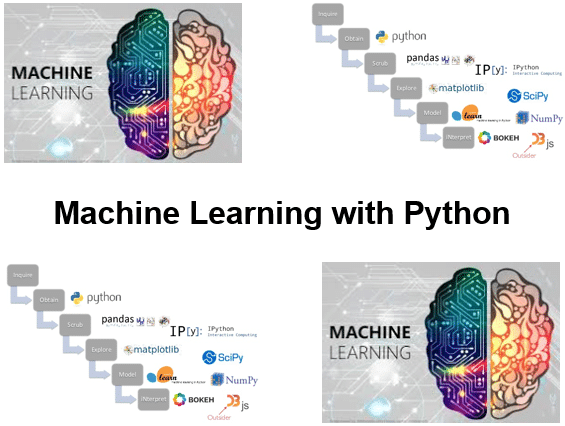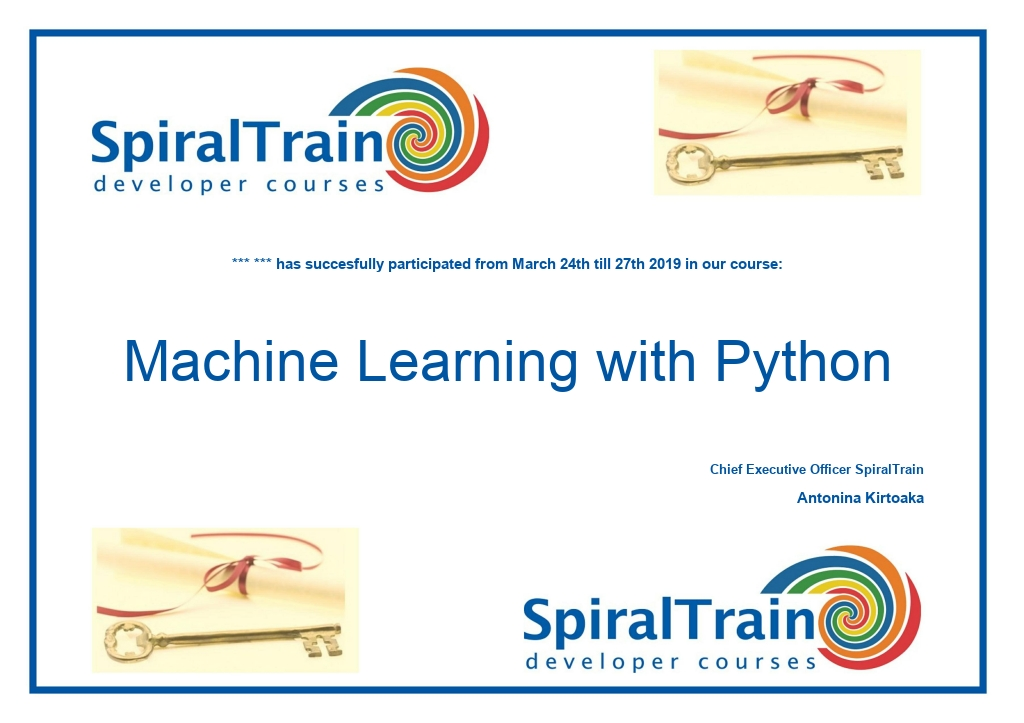# Course Machine Learning with Python

In the course Machine Learning with Python participants learn how to implement machine learning algorithms using Python and the Scikit-learn library. Scikit-learn is largely written in Python and makes extensive use of Numpy for high-performance linear algebra and array operations.

Region:
4 days
€ 2650
• Content
• Training
• Modules
• General
General
• Reviews
• Certificate
• ## Course Machine Learning with Python : Content

### Machine Learning Intro

The Machine Learning with Python course starts with an overview of the basic concepts of Machine Learning in which models are made on the basis of supplied data. The difference is explained between Supervised and Unsupervised Learning.

### Scikit-learn Library

Subsequently the libraries that form the foundation behind Machine Learning with Scikit-learn such as Numpy, Pandas, MatPlotLib and Seaborn are discussed. In the basic architecture of Scikit-learn, the data is split into a feature matrix and a target array. Also treated is how a model is trained with a training set and then compared to a test set with the Estimator API.

### Feature Handling

The course Machine Learning with Python also includes Feature Engineering. This discusses how to deal with categorical features, text features, image features and derived features. And the use of features pipelines is also explained.

### Regressions

After a treatment of the Naive Bayes theorem with Naive Bayes classifiers and the models based on them, Linear and Logistic regression are discussed. Specialist versions such as Polynomial Regression, Ridge Regression and Lasso Regularization are also covered.

### Classifications

Then the course Machine Learning with Python pays attention to different variants of Machine Learning algorithms that are based on classification. Support Vector Machines and Decision Trees are discussed here.

### Unsupervised Learning

Finally the course Machine Learning with Python deals with Principal Component Analysis as an example of an unsupervised learning algorithm. Dimensionality Reduction is then treated as well.

• ## Course Machine Learning with Python : Training

### Audience Course Machine Learning with Python

The course Machine Learning with Python is intended for data analysts who want to use Python and the Python libraries in Data Analysis projects.

### Prerequisites training Machine Learning with Python

To participate in this course knowledge of and experience with any programming language or package such as SPSS, Matlab or VBA is desirable. The course starts with a discussion of the principles of the Python programming language.

### Realization Course Machine Learning with Python

The theory is discussed on the basis of presentation slides. Illustrative demos clarify the concepts. The theory is interchanged with exercises. The Anaconda distribution with Jupyter notebooks is used as a development environment. Course times are from 9:30 to 16:30.

### Official Certificate Machine Learning with Python

After successful completion of the course, participants receive an official Machine Learning certificate with Python.• ## Course Machine Learning with Python : Modules

### Module 3 : Scikit-learn Library

What is Machine Learning?
Building Models of Data
Model Based Learning
Tunable Parameters
Supervised Learning
Labeling Data
Discrete Labels
Continuous Labels
Classification and Regression
Unsupervised Learning
Data Speaks for Itself
Clustering and Dimensionality Reduction
Numpy Arrays
NumPy Data Types
Pandas Data Frames
Inspect Data
Operations on Data
Missing Data
Pandas Series
Pandas Indexes
Time Series
MatplotLib
Plotting with Pandas
Seaborn Library
Data Representation
Estimator API
Features Matrix
Target Array
Seaborn Visualization
Model Classes
Choosing Hyperparameters
Model Validation
Fit and Predict Method
Label Predicting
Training and Testing Set
Transform Method

### Module 6 : Linear Regression

Categorical Features
Vectorization
Text and Image Features
Derived Features
Handling Missing Data
Imputation of Missing Data
Feature Pipelines
Polynomial Basis Functions
Gaussian Basis Functions
Regularization
Naive Bayes Classifiers
Applicability
High Dimensional Datasets
Bayes’s Theorem
Generative Models
Gaussian Naive Bayes
Probabilistic Classification
predict_proba Method
Multinomial Naive Bayes
Confusion Matrix
When to Use Naive Bayes
Slope and Intercept
LinearRegression Estimator
coef_ and intercept_ Parameter
Multidimensional Linear Models
Basis Function Regression
Polynomial Regression
PolynomialFeatures Transformer
Gaussian Basis Functions
Overfitting
Ridge Regression
Lasso Regularization

### Module 9 : Principal Components

Discriminative Classification
Maximizing the Margin
Linear Kernel
C Parameter
Support Vectors
SVM Visualization
Kernel SVM
Kernel Transformation
Kernel Trick
Softening Margins
Ensemble Learner
Creating Decision Trees
DecisionTree Classifier
Overfitting Decision Trees
Ensembles of Estimator
Random Forests
Parallel Estimators
Bagging Classifier
Random Forest Regression
RandomForest Regressor
Non Parametric Model
PCA Unsupervised Learning
Principal Axes
Demonstration Data
Affine Transformation
Components
Explained Variance
Dimensionality Reduction
Inverse Transformation
Explained Variance Ratio
PCA as Noise Filtering
• ## Course Machine Learning with Python : General